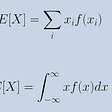# Dijkstra’s algorithm

DIJKSTRA’S ALGORITHM

Dijkstra’s algorithm is an algorithm for finding out the shortest path in a graph between the nodes basically given a source node in the graph the algorithm finds the shortest path between that node and every other node present in the graph, it can also be used for finding the shortest path between a single node to a single destination node and the algorithm stops as soon as the shortest path is found/ determined

For example- Dijkstra’s algorithm is used to find the shortest path between one city and all other cities where cities are representation of nodes in a graph.

Dijkstra’s algorithm uses a data structure for storing the partial solution sorted by distance from the start while the original shortest pathfinding algorithm uses a minimum-priority queue and runs with the time complexity of O((V +E) log(V)) (V is the number of nodes and E is the number of edges), It can also be implemented using an array but the time complexity for the same is O(V²)

this is the fastest shortest pathfinding algorithm for the directed graphs with unbounded nonnegative edge weights.

HISTORY

The algorithm was developed by EDSGER W. DIJKSTRA in 1956 when he was working at the mathematical center in Amsterdam as a programmer.

TIME COMPLEXITY

the time complexity of O((V +E) log(V)) (V is the number of nodes and E is the number of edges), It can also be implemented using an array but the time complexity for the same is O(V²)

this is the fastest shortest pathfinding algorithm for the directed graphs with unbounded nonnegative edge weights.

WORKING

Let the starting node be called the initial node, Dijkstra’s algorithm will initially start with infinite distances and will try to improve them step by step

1. Mark all nodes unvisited, and create a set of all the unvisited nodes that are called the unvisited set.

2. Assign a tentative distance to each node: set it zero for the initial node and to infinity for all the other nodes, the distance of a node V is the length of the shortest path discovered so far between node V and the initial node all the other distances are set to infinity at the start.

3. Consider all of the unvisited neighbors of the current node and calculate their distances through the current node. Compare the newly calculate the distance to the current assigned value and assign the smaller one.

4. After traversing through all the unvisited neighbors of the current node mark the current node as the visited node and remove it from the unvisited set. a visited node will never be checked again.

5. If the destination node has been marked visited and if the smallest distance among the nodes in the unvisited set is infinity then STOP the algorithm has finished.

PSEUDOCODE

--

--

--

## More from Aryan goel

just another tech enthusiast

Love podcasts or audiobooks? Learn on the go with our new app.

## Probability distributions## The most beautiful math equation ever## Continuity: An Ode to the Well Behaved## Hypothesis Testing: A Way to Accept or Reject Your Hypothesis Using the p-value## What Do You Expect the Mean to be equal to?## Measurement in Quantum Mechanics## Aryan goel

just another tech enthusiast

## Introducing: VeendHQ Latest Features## What Is Optical Character Recognition?